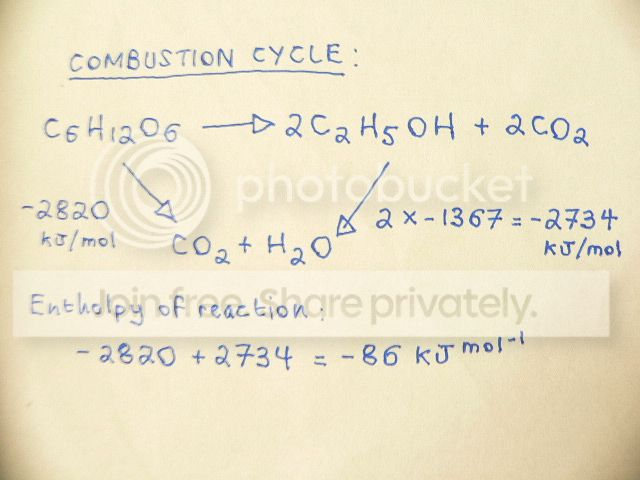###Topic: Enthalpy of Reaction for Fermentation of Glucose  (Read 473 times)

0 Members and 1 Guest are viewing this topic.

####happychappie1700

• New Member
•• Posts: 8
• Mole Snacks: +0/-0##### Enthalpy of Reaction for Fermentation of Glucose
« on: February 19, 2019, 10:15:52 AM »
Dear Colleagues,

The following question asks me: Calculate the Enthalpy of Reaction for ( using Hess's Law ):

C6H12O6  ----->2C2H5OH+2CO2

Using the following data ( Enthalpy of Combustion ): Glucose=-2820 Ethanol -1367 The answer is:The answer then states that there is a linear method available for obtaining the same answer:
ΔrH=ΣΔcHreactant-ΣΔcHreactant

Which is : -2820 - ( 2 x -1367 ) = - 86 KJ mol

My confusion is: if the question is asking for the Enthalpy of Reaction ( Delta H ) - assuming its for the whole reaction, then why is the other product CO2 not included in the calculation ? ( why is only 2C2H5OH included in the calculation ? ).

####Babcock_Hall

• Chemist
• Sr. Member
•• Posts: 3623
• Mole Snacks: +234/-16##### Re: Enthalpy of Reaction for Fermentation of Glucose
« Reply #1 on: February 19, 2019, 12:25:55 PM »
I would try writing out each chemical reaction and adding them as appropriate.

####mjc123

• Chemist
• Sr. Member
•• Posts: 1532
• Mole Snacks: +219/-9##### Re: Enthalpy of Reaction for Fermentation of Glucose
« Reply #2 on: February 19, 2019, 12:41:16 PM »
What do you think the combustion enthalpy of CO2 is? Can you combust CO2?

####happychappie1700

• New Member
•• Posts: 8
• Mole Snacks: +0/-0##### Re: Enthalpy of Reaction for Fermentation of Glucose
« Reply #3 on: February 19, 2019, 04:04:36 PM »
What do you think the combustion enthalpy of CO2 is? Can you combust CO2?

I presume you can't combust Carbon Dioxide, but why is Carbon Dioxide taken into account in the following question / equation:

Calculate the standard enthalpy of combustion of Methanol:

2CH3OH + 3O2 -----> 4CO2+2H2O

Using Enthalpy of Formation Data:
CH3OH = -238.6 KJ/mol
O2 = 0
CO2 = -393.5 KJ/mol
H20 = -241.8 KJ/mol

Equation = ΔrH=ΣΔfHproducts -ΣΔfHreactants
= [ 4 (-393.5) + 2 (-241)] - [2 (-238.6) + (0)] = -1277 KJ / Mol

Why is the CO2 figure of -393.5 included in this calculation ( why is it not ignored as in the first question about the fermentation of glucose )?

####mjc123

• Chemist
• Sr. Member
•• Posts: 1532
• Mole Snacks: +219/-9##### Re: Enthalpy of Reaction for Fermentation of Glucose
« Reply #4 on: February 20, 2019, 08:37:26 AM »
Because CO2 has an enthalpy of formation, but not an enthalpy of combustion.
Consider the equations
2C + 4O2 + 4H22CH3OH + 3O2 ΔH1 = 2ΔHfCH3OH
2C + 4O2 + 4H22CO2 + 4H2O   ΔH2 = 2ΔHfCO2 + 4ΔHfH2O
2CH3OH + 3O22CO2 + 4H2O ΔH3 = ΔH2 - ΔH1 = 2ΔHcombCH3OH
(note you balanced the equation wrongly, and the final answer has to be divided by 2)
You can see, I hope, how the enthalpy of formation of CO2 must be included here.
But consider the right hand side of your combustion cycle:
2C2H5OH + 2CO2 + 6O2{4CO2 + 6H2O} + 2CO2
The 2CO2 on the LHS is unchanged, so there is no enthalpy change associated with it.